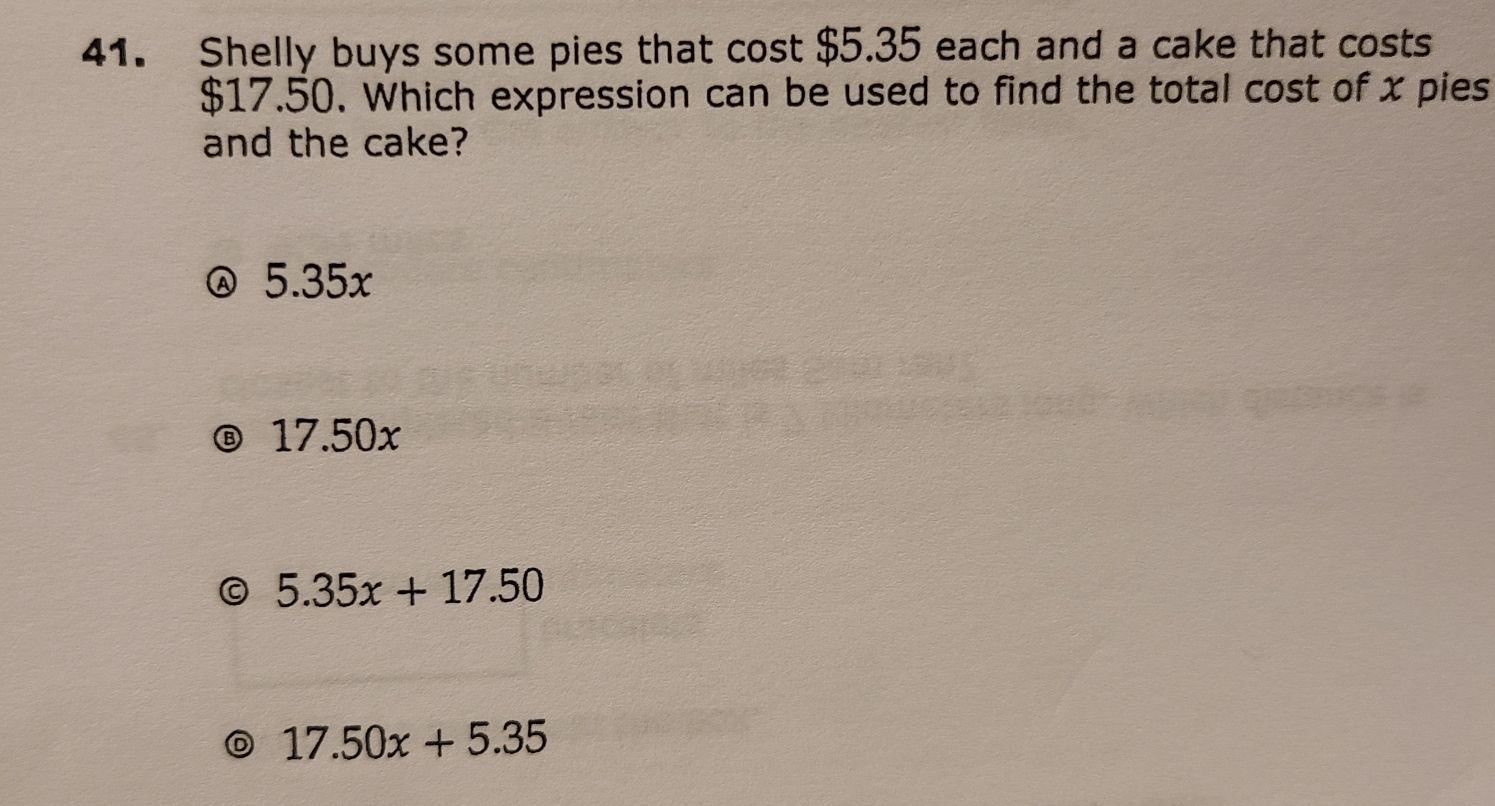### ¿Todavía tienes preguntas de matemáticas?

Pregunte a nuestros tutores expertos
Algebra
Pregunta41. Shelly buys some pies that cost $$\ 5.35$$ each and a cake that costs $$\ 17.50$$ . Which expression can be used to find the total cost of $$x$$ pies and the cake?

(A) $$5.35 x$$

(B) $$17.50 x$$

(C) $$5.35 x + 17.50$$

(D) $$17.50 x + 5.35$$

C. $$5.35x+ 17.50$$